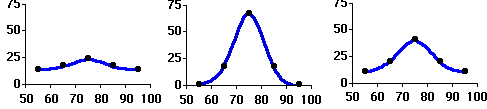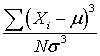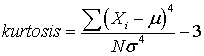### Why should I choose AnalystNotes?

AnalystNotes specializes in helping candidates pass. Period.

##### Subject 10. Kurtosis in Return Distributions
We've just discussed skewness, which refers to the deviation from a normal distribution due to asymmetry. A distribution may deviate from the normal distribution by being more or less peaked than a normal distribution. Kurtosis is based on the size of a distribution's tails. It is the statistical measure that tells us when a distribution is more or less peaked than a normal distribution.

• Distributions with small tails (that is, less peaked than normal) are called "platykurtic." If a return distribution has more returns with large deviations from the mean, it is platykurtic.
• Distributions with relatively large tails (that is, more peaked than normal) are called "leptokurtic." If a return distribution has more returns clustered closely around the mean, it is leptokurtic.
• A distribution with the same kurtosis as the normal distribution is called "mesokurtic."Kurtosis is critical in a risk management setting. Most research on the distribution of securities returns has shown that returns are not normal. Actual securities returns tend to exhibit both skewness and kurtosis (sounds like fungus!). Skewness and kurtosis are critical for risk management because if securities returns are modeled after a normal distribution, predictions from those models will take into consideration the potential for extremely large negative outcomes. In fact, most risk managers put very little emphasis on the mean and standard deviations of a distribution and focus more on the distribution of returns in the tails of the distribution, since this is where the risk is.

To calculate skewness (when N is large):where μ is the mean and σ is the standard deviation.

The normal distribution has a skew of 0, since it is a symmetric distribution.
If skewness is positive, the average magnitude of positive deviations is larger than the average magnitude of negative deviations.

To calculate excess kurtosis (when N is large):The kurtosis equals excess kurtosis + 3.

For all normal distributions, kurtosis is equal to 3; excess kurtosis is equal to 0.

A leptokurtic distribution has an excess kurtosis greater than 0, and a platykurtic distribution has an excess kurtosis less than 0.

Learning Outcome Statements

j. explain skewness and the meaning of a positively or negatively skewed return distribution;

k. describe the relative locations of the mean, median, and mode for a unimodal, nonsymmetrical distribution;

l. explain measures of sample skewness and kurtosis;

CFA® 2021 Level I Curriculum, , Volume 1, Reading 7

User Comment
chandos PLATY, LEPTO, MESO
cwrolfe NINA, PINTA, SANTA MARIA
AusPhD alpha, beta, gamma
achu "lepto" leaps OVER normal kurtosis. platy is thus under the normal kurtosis.
kurtosis = formula "+0" The -3 constant is added to calcluate XS kurtosis.
GeorgeC Remember "platy" is like "plateau" which is flat - thus the platykurtic distribution is flatter.
mordja Veni, Vidi, Vici
TammTamm Uno, Dos, Tres
ravdo ep, dog, dig.
alyl21 Distributions with small tails (that is, less peaked than normal) are called "platykurtic." If a return distribution has more returns with large deviations from the mean (and thus has fatter tails), it is platykurtic.

Note the error in GeorgeC's comment: Platykurtic is less peaked and has thinner tails, not fatter tails. Check CFA curriculum
Criticull I second alyl21's comment since the distribution is less peaked, but still must have total probability sum to one between the pdf and the horizontal axis.
jack1jack I agree with alyl21. leptokurtic return distribution is has the fatter tail.
anastasiya The formulas posted here are valid only if N gets large - both for the skewness and the kurtosis. The CFA textbook gives broader and more complex formulas but there's no way to memorize them.
rhardin Way to remember: Leptokurtic needs liposuction because it's tails are fat!
ciji A platykurtic distribution is like a platypus
jpducros These definitions seems basic but need special attention since it is quite easy (for me at least) to make a mistake. Confusion is even more probable when you'll work on t-distribution and degree of freedom. The higher the df, the higher the peak, but the thinner the tails (different from what we've seen above). In other words, don't confuse degree of freedom and lepto/platy kurdic. Hope it helps.
Kbrooks80 An electron is a lepton. For leptokurtic just think of the mean as a conductive wire running vertically through the distribution that the electrons gravitate toward.
dipu617 ek, dui, tin .....
rockstarchuckie een, twee, drie
Creep apple, cat, schizophrenic
RamaG lepto = normality takes a leap
playto = normality is played down
schweitzdm Notice that the LOS does not say that we need to know to calculate kurtosis. Need to be able to explain though.
sahilb7 L for Lepto - L for Long
P for Platy - P for Plateau
M for Meso - M for Middle
ravinram For further information on this topic, pls visit the website:
vassarstats.net/textbook/ch2pt1.html
forry9er PLATYKURTIC distributions have FLAT tails like a PLATYPUS. Didn't anyone take Latin?
ashish100 these guys are losing their mind studying for this
khalifa92 you'ven't seen anything yet.
fin_guy I don't get it. They say kurtosis is "peaky" or "flatty" of returns. Then there is "kurtosis risk". Why is "peaky" or "flatty" called "risky"?
tejerius pim, pam , pum, bocadillo de atún
ocanas XBOX, NINTENDO, SONY